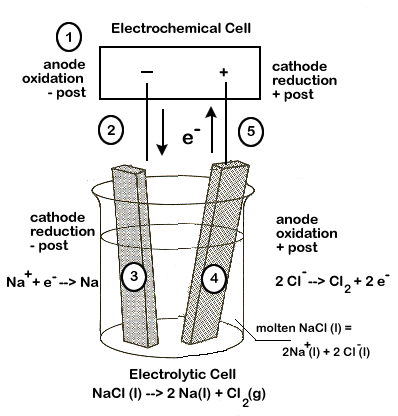# 3.2 Electrolysis of Molten Sodium Chloride

If we look at the latin roots of the word "electrolysis" we learn that it means, essentially, to "break apart" (lysis) using electricity.

Our first example of electrolytic cell will examine how an electric current can be used to break apart an ionic compound into its elements.

The following equation represents the breaking apart of $$\ce{NaCl}$$:

$$\ce{2NaCl(l) -> 2Na(l) + Cl2(g)}$$

The half-reactions involved in this process are:

 E° reduction $$\ce{2Na+(aq) + 2e^{-} -> 2Na(s)}$$ -2.71 V oxidation $$\ce{2Cl^{-}(l) -> Cl2(g) + 2e^{-}}$$ -1.36V net voltage required - 4.07V

Notice that a negative voltage (-4.07V) results when we add up the half-reactions. This tells us that the overall reaction will NOT be spontaneous, and a minimum of 4.07 volts will be required for this reaction to occur.

As we shall see, our set-up will have a number of similarities to our electrochemical cells. We will need electrodes and an electrolyte to carry the electric current.

In our $$\ce{NaCl}$$ example, the electrodes will simply carry the current, but otherwise not be directly involved in the reaction. The electrolyte will be the actual molten (melted) $$\ce{NaCl}$$. The electrodes and electrolyte are both required to carry the electric current. Molten $$\ce{NaCl}$$ must be used because solid ionic compounds do not carry an electric charge.

Some key differences with an electrochemical cell set-up:

• the two half-reactions are not separated by a salt bridge
• an electrochemical cell (or other source of electric current) will be required

Other important items to note:

• The anode of the electrolytic cell is the site of oxidation and the cathode is the site of reduction, just as in an electrochemical cell.
• In an electrochemical cell, the anode is negative and cathode positive, but this is reversed in the electrolytic cell - the anode is positive and the cathode is negative.

Carefully study the diagram of our set-up, taking special care to trace the path of the electrons. Unless electrons make a complete circuit, a reaction will not occur.

1. Electrons are "produced" in the battery at the anode, the site of oxidation.
2. The electrons leave the electrochemical cell through the external circuit.
3. These negative electrons create a negative electrode in the electrolytic cell which attracts the positive $$\ce{Na+}$$ ions in the electrolyte. $$\ce{Na+}$$)
4. Meanwhile the negative $$\ce{Cl-}$$ become attracted to the positive electrode of the electrolytic cell. At this electrode chlorine is oxidized, releasing electrons ($$\ce{2Cl^{-}(l) -> Cl2(g) + 2e^{-}}$$)
5. These electrons travel through the external circuit, returning to the electrochemical cell.